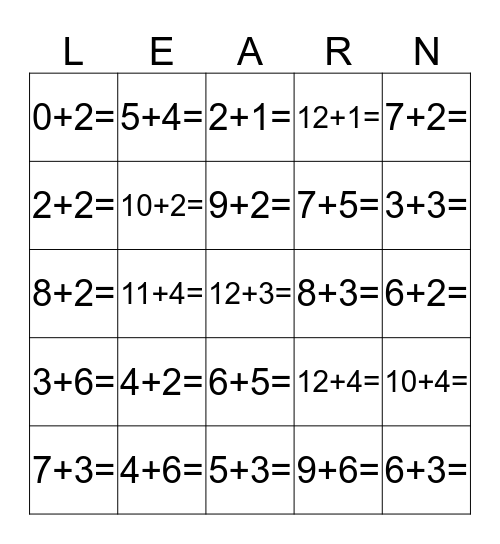# Let's Practice Our TablesThis bingo card has 79 words: 0+1=, 1+1=, 2+1=, 3+1=, 4+1=, 5+1=, 6+1=, 7+1=, 8+1=, 9+1=, 10+1=, 11+1=, 12+1=, 0+2=, 1+2=, 2+2=, 3+2=, 4+2=, 5+2=, 6+2=, 7+2=, 7+2=, 8+2=, 9+2=, 10+2=, 11+2=, 12+2=, 0+3=, 1+3=, 2+3=, 3+3=, 4+3=, 5+3=, 6+3=, 7+3=, 8+3=, 9+3=, 10+3=, 11+3=, 12+3=, 0+4=, 1+4=, 2+4=, 3+4=, 4+4=, 5+4=, 6+4=, 7+4=, 8+4=, 9+4=, 10+4=, 11+4=, 12+4=, 0+5=, 1+5=, 2+5=, 3+5=, 4+5=, 5+5=, 6+5=, 7+5=, 8+5=, 9+5=, 10+5=, 11+5=, 12+5=, 0+6=, 1+6=, 2+6=, 3+6=, 4+6=, 5+6=, 6+6=, 7+6=, 8+6=, 9+6=, 10+6=, 11+6= and 12+6=.

⚠ This card has duplicate items: 7+2= (2)

## Play Online

Share this URL with your players:For more control of your online game, create a clone of this card first.

## Probabilities

With players vying for a you'll have to call about __ items before someone wins. There's a __% chance that a lucky player would win after calling __ items.

Tip: If you want your game to last longer (on average), add more unique words/images to it.